Breaking News

#### Select Language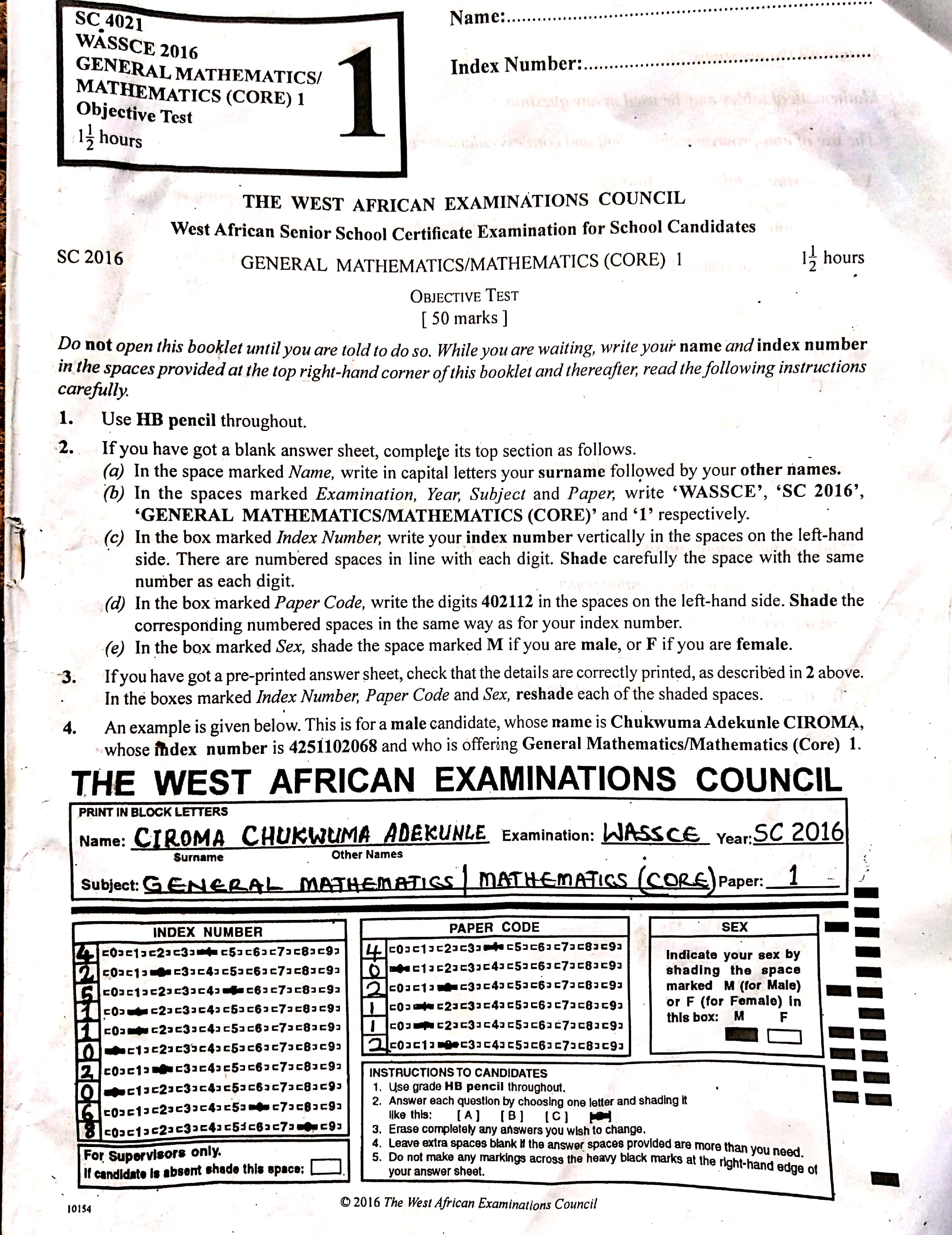These are 2016 WAEC General Mathematics Past Questions And Answers

Note:

( ^ ) means superscript or raise to the power of..

( _ ) means subscript

1. An object is 6m away from the base of a mast. The angle of depression of the object from the top of the mast is 50°, Find, correct to 2 decimal places, the height of the mast

A) 8.60m

B) 7.51m

C) 7.15m

D) 1.19m

Explanation:

tan 50° = h/6

h = 6 tan 50°

= 6 × 1.1917

= 7.1505

= 7.15

2. The relation y = x²+ 2x + k passes through the point (2, 0). Find the value of k

A) 8

B) -2

C) -4

D) -8

Explanation:

y = x² + 2x + k at point(2,0) x = 2, y = 0

0 = (2)2 + 2(20 + k)

0 = 4 + 4 + k

0 = 8 + k

k = -8

3. If 23_x = 32_5, find the value of x

A) 7

B) 6

C) 5

D) 4

Explanation:

23_x = 32_5

2 × x¹ + 3 × x⁰ = 3 × 5¹ + 2 × 5⁰

= 2x + 3 = 15 + 2

2x + 3 = 17

2x = 17 – 3

2x = 14

x = 14/2

x = 7

4. The curved surface area of a cylinder 5cm high is 110cm². Find the radius of its base

[ Take π = 22/7 ]

A) 2.6cm

B) 3.5cm

C) 3.6cm

D) 7.0cm

Explanation:

Curved surface area of cylinder = 2πrh

110 = 2 × 22/7 × r × 5

r = 110×7/44×5

= 3.5cm

5. Make s the subject of the relation:

p = s + sm²/nr

A) s = nrp/nr+m²

B) s = nr + m²/mrp

C) s = nrp/mr + m²

D) s = nrp/nr + m²

Explanation:

p = s + sm²/nr

p = s + ( 1 + m²/nr)

p = s (1 + nr + m²/nr)

nr × p = s (nr + m²)

s = nrp/nr+m²

6. Simplify:(10√3 /√5 − √15)²

A) 75.00

B) 15.00

C) 8.66

D) 3.87

7. The distance, d, through which a stone falls from rest varies directly as the square of the time, t, taken. If the stone falls 45 cm in 3 seconds, how far will it fall in 6 seconds?

(more at ngstudents.com)

A) 90 cm

B) 135 cm

C) 180 cm

D) 225 cm

Explanation:

d α t²

d = t² k

where k is a constant. d = 45cm, when t = 3s; thus 45 = 3² x t

k = 45/9 = 5

thus equation connecting d and t is d = 5t²

when t = 6s, d = 5 x 6²

= 5 x 36

= 180cm

8. Which of following is a valid conclusion from the premise. “Nigeria footballers are good footballers”?

A) Joseph plays football in Nigeria therefore he is a good footballer

B) Joseph is a good footballer therefore he is a Nigerian footballer

C) Joseph is a Nigerian footballer therefore he is a good footballer

D) Joseph plays good football therefore he is a Nigerian footballer

Explanation:

From the venn diagram, Nigeria footballers from a subset of good footballers.

9. On a map, 1 cm represent 5 km. Find the area on the map that represents 100km².

A) 2cm²

B) 4cm²

C) 8cm²

D) 16cm²

Explanation:

On a map, 1 cm represents 5 km. Then it follows that 1 cm² represents 25 km². A cm² represents 100 km². By apparent cross-multiplication, 1 cm² x 100 km² = A cm² x 25 km²

therefore A = 100/25

= 4 cm²

10. Simplify; 3^(n−1) × 27^(n+1) / 81^n

A) 3^2n

B) 9

C) 3^n

D) 3^(n + 1)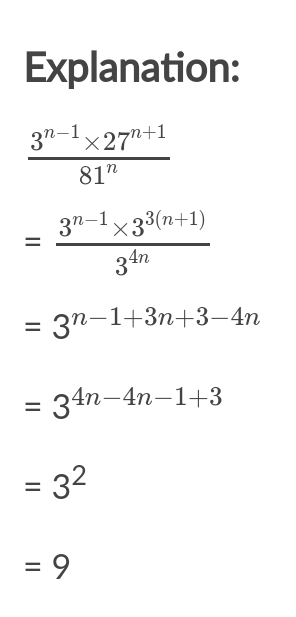11. What sum of money will amount to D10,400 in 5 years at 6% simple interest?

Options

A) D8,000.00

B) D10,000.00

C) D12,000.00

D) D16,000.00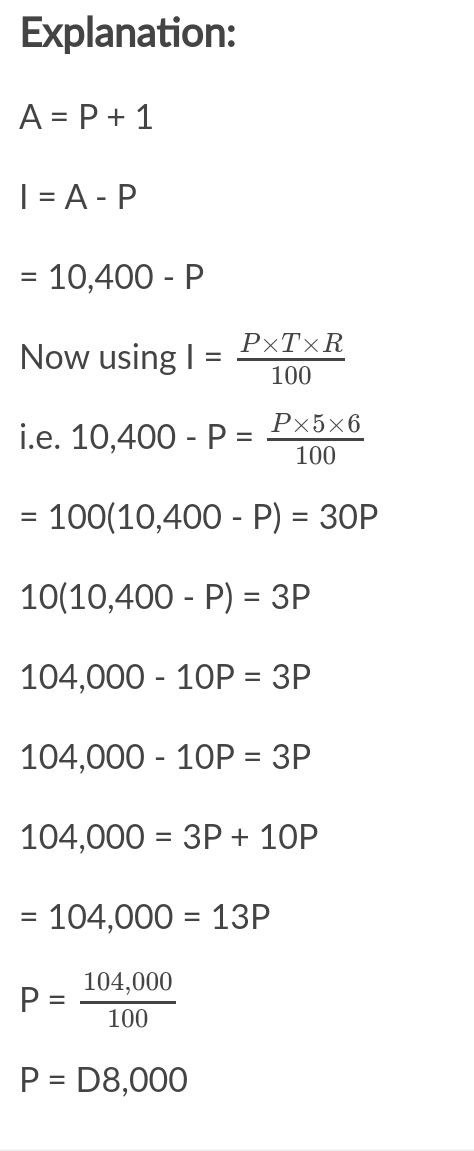12. The roots of a quadratic equation are 4/3 and -3/7. Find the equation

A) 21x² – 19x – 12 = 0

B) 21x² + 37x – 12 = 0

C) 21x² – x + 12 = 0

D) 21x² + 7x – 4 = 0

Explanation:

Let x = 4/3, x = -3/7

Then 3x = 4, 7x = -3

3x – 4 = 0, 7x + 3 = 0

(3x – 4)(7x + 3) = 0

21x² + 9x – 28x – 12 = 0

21x² – 19x – 12 = 0

13. Find the values of y for which the expression (y²−9y+18)/(y²+4y−21) is undefined

A) 6, –7

B) 3, -6

C) 3, -7

D) -3, -7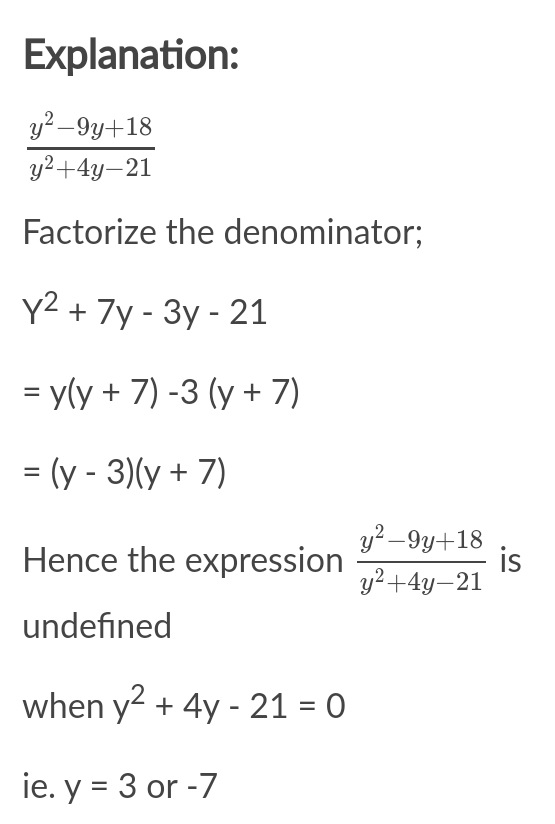14. Given that 2x + y = 7 and 3x – 2y = 3, by how much is 7x greater than 10?

A) 1

B) 3

C) 7

D) 17

Explanation:

2x + y = 7…(1)

3x – 2y = 3…(2)

From (1), y = 7 – 2x for y in (2)

3x – 2(7 – 2x) = 3

3x – 14 + 4x = 3

7x + 3 + 14 = 17

x = 17/7

Hence, 7 x 17/7

= 17 – 10

= 7

15. Simplify; 2/1−x − 1/x

A) x+1/x(1−x)

B) 3x−1/x(1−x)

C) 3x+1/x1−x)

D) x–1x/x(1−x)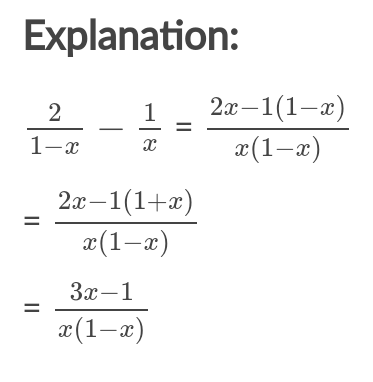16. Factorize; (2x + 3y)² – (x – 4y)²

A) (3x – y)(x + 7y)

B) (3x + y)(2x – 7y)

C) (3x + y)(x – 7y)

D) (3x – y)(2x + 7y)

Explanation:

(2x + 3y)² – (x – 4y)²

= (2x + 3y)(2x + 3y) – (x – 4y)(x – 4y)

= 4x² + 12xy + 9y² – (x² – 8xy+ 16y²)

= 4x² + 12xy + 19y² – x² + 8xy – 16y²

= 3x² + 20xy – 7y²

= 3x² + 21xy – xy – 7y²

= 3x(x + 7y) – y(x + 7y)

= (3x – y)(x + 7y)

17. The volume of a pyramid with height 15 cm is 90 cm³. If its base is a rectangle with dimension x cm by 6 cm, find the value of x

A) 3

B) 5

C) 6

D) 8

Explanation:

Volume of pyramid = 1/3lbh

90 = 1/3 × x × 6 × 15

x = 90×33/6×15

= 3

A straight line passes through the points P( 1,2) and Q(5,8). Use this information to answer questions 18 and 19.

18. Calculate the gradient of the line PQ

A) 3/5

B) 2/3

C) 3/2

D) 5/3

Explanation:

Let: (x¹, y¹) = (1, 2)

(x², y²) = (5, 8)

The gradient m of ¯PQ is given by

m = y_2–y_1/x_2−x_1

=8−2/5−1

= 6/4

= 3/2

19. Calculate the length PQ.

A) 4√11

B) 4√10

C) 2√17

D) 2√13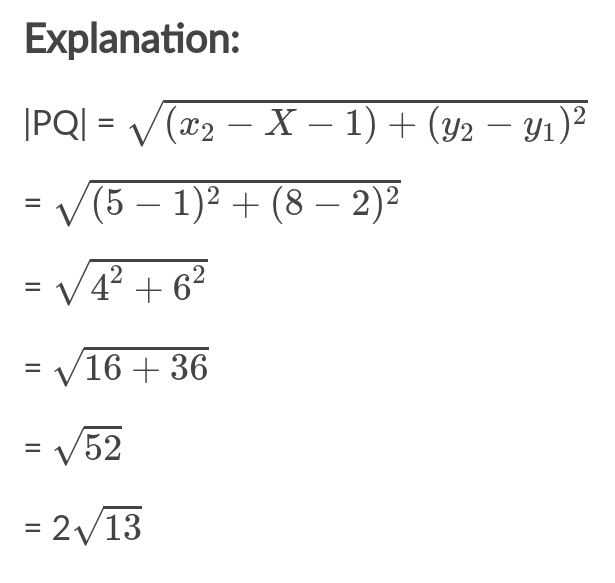20. If cos θ = x and sin 60° = x + 0.5 , 0° < θ < 90°, find, correct to the nearest degree, the value of θ

A) 66°

B) 67°

C) 68°

D) 69°

Explanation:

sin 60° = x + 0.5 0°=(given)

0.8660 = x + 0.5

0.8660 – 0.5 = x

x = 0.3660

cosθ= x(given)

cosθ= 0.3660

Hence, θ= cos^-1(0.3660)

= 68.53°

= 69° (nearest degree)

21. The table shows the ages of students in a club. How many students are in the club?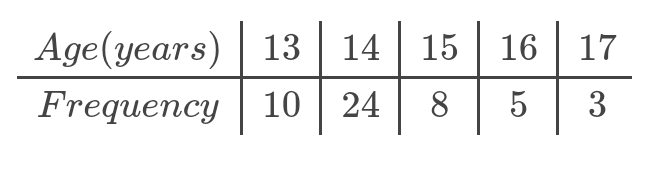A) 50

B) 55

C) 60

D) 65

Explanation:

Number of students in the club is

10 + 24 + 8 + 5 + 3 = 50

22. Find the median ageA) 13

B) 14

C) 15

D) 16

Explanation:

Median = N/2 th age

= 50/2 th age

= 25th age

= 14 years

18.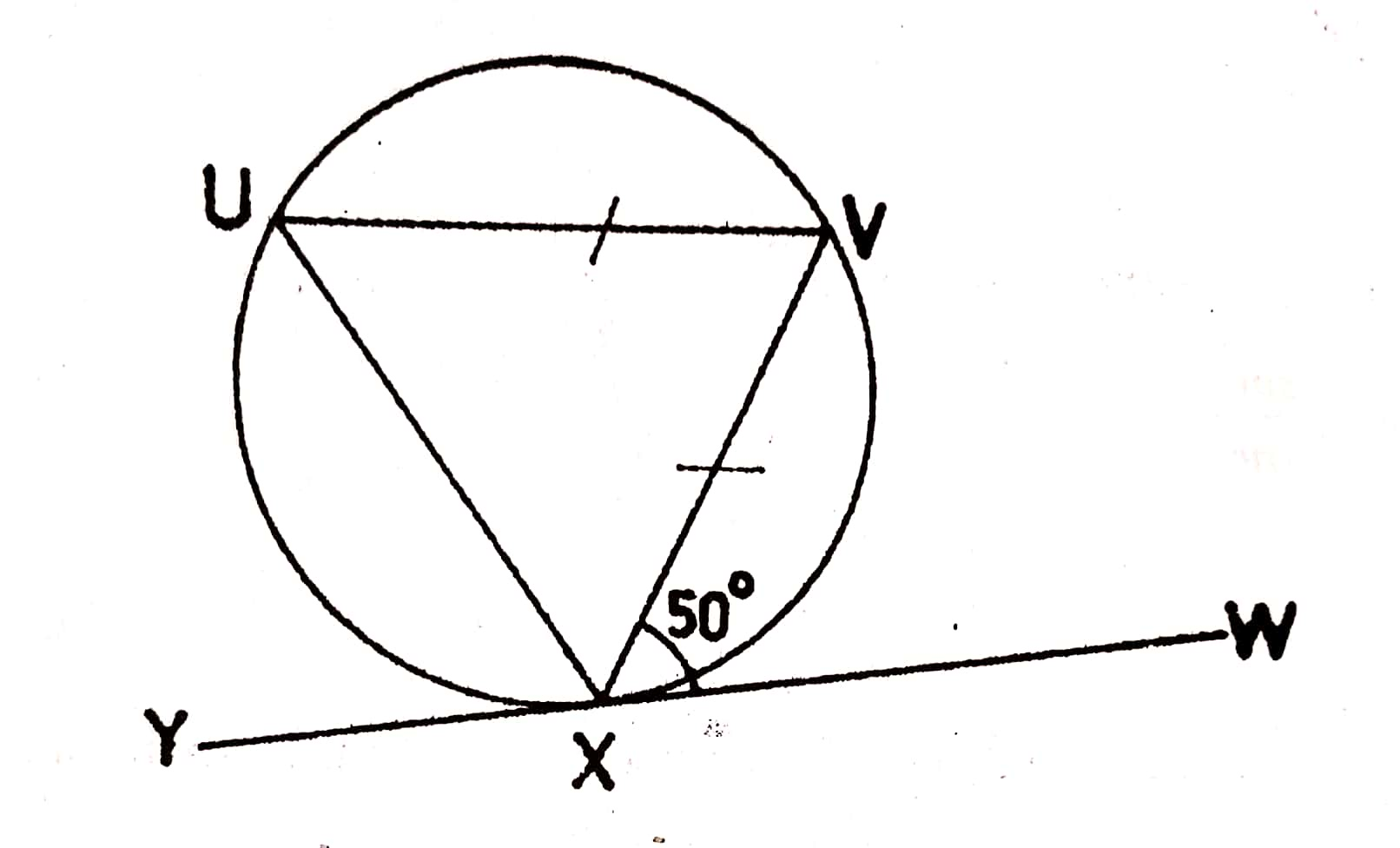In the diagram, ¯YW is a tangent to the circle at X, |UV| = |VX| and <VXW = 50°. Find the value of <VXY.

A) 70°

B) 80°

C) 105°

D) 110°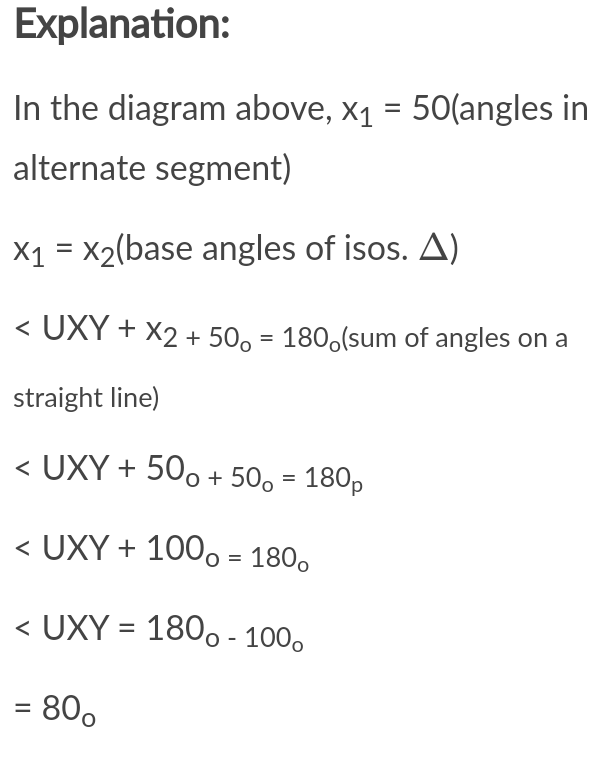Question 24

24.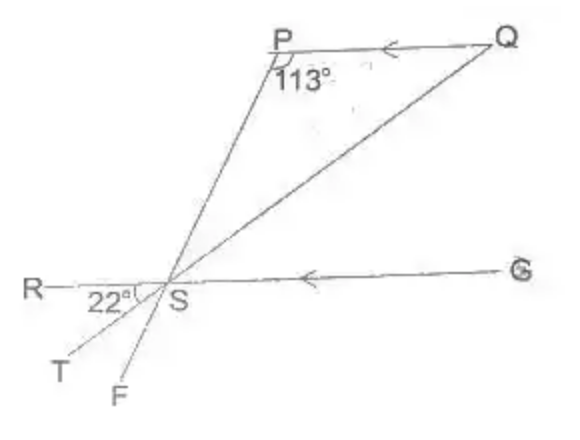In the diagram, ¯PF, ¯QT, ¯RG intersect at S and PG||RG. If < SPQ = 113° and < RST = 22°, find < PSQ.

A) 22°

B) 45°

C) 67°

D) 89°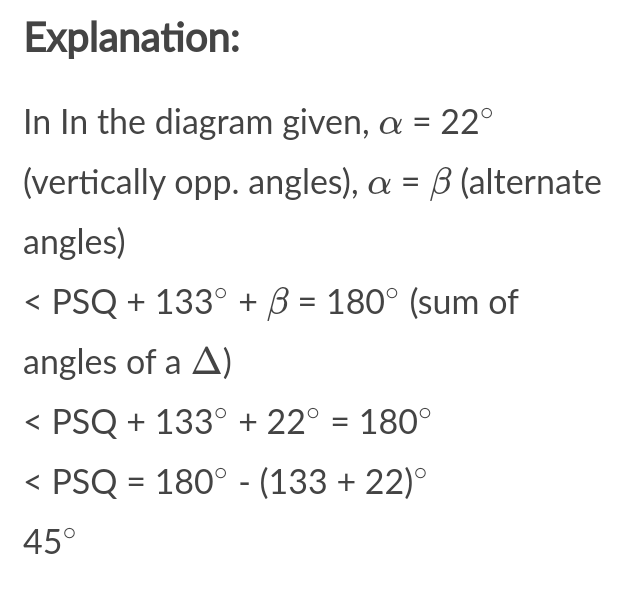25.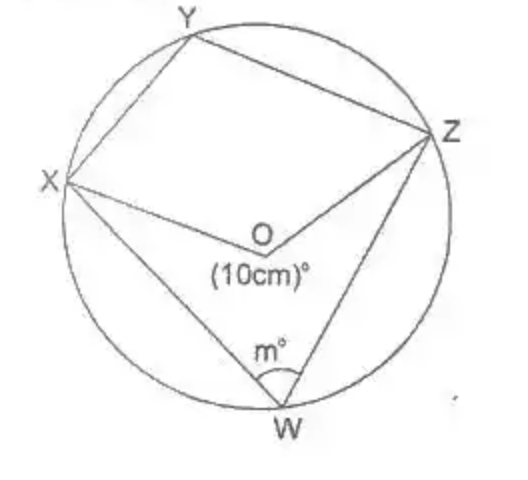In the diagram, O is the centre of the circle, < XOZ = (10m)° and < XWZ = m°. Calculate the value of m.

A) 30

B) 36

C) 40

D) 72

Explanation:

In the diagram above, α = 2m° (angle at centre = 2 x angle at circumference)

α + 10m° = 360° (angle at circumference)

α + 10m° = 360° (angles round a point)

2mo + 10m° = 360°

12m° = 360°

m° = 360°/12

= 30°

26. Kweku walked 8m up to slope and was 3m above the ground. If he walks 12m further up the slope, how far above the ground will he be?

A) 4.5 m

B) 6.0 m

C) 7.5 m

D) 9.0 m

Explanation:

By similar triangles, 8/3 = 8+12/h

8/3=20/h

h = 3×20/8

= 7.5m

27.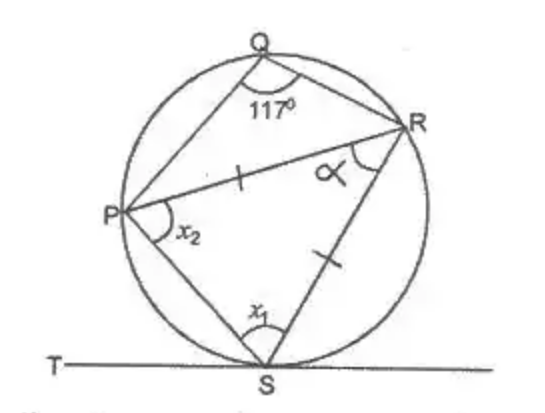In the diagram, TS is a tangent to the circle at S. |PR| = |RS| and < PQR = 177°. Calculate < PST.

A) 54°

B) 44°

C) 34°

D) 27°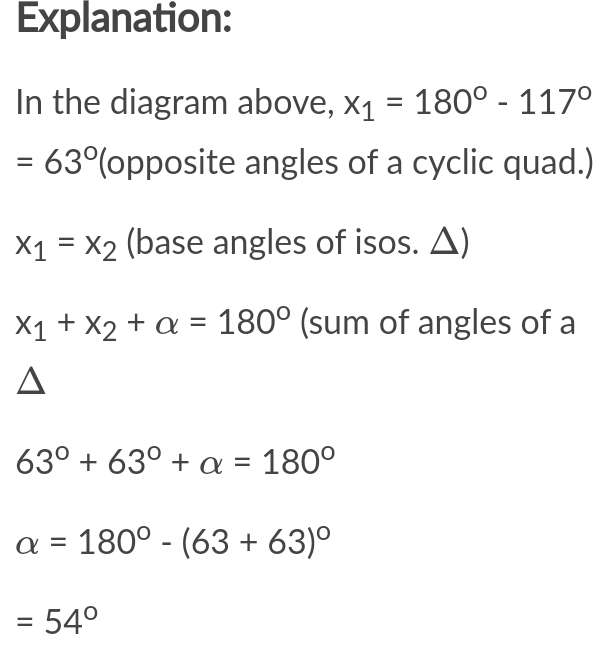28.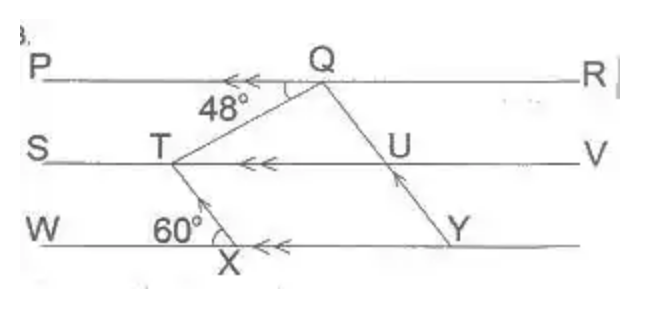In the diagram, PR||SV||WY|, TX||QY|, < PQT = 48° and < TXW = 60°. Find < TQU.

A) 120°

B) 108°

C) 72°

D) 60°

Explanation:

In the diagram, < TUQ + 60°(corresp. angles)

< QTU = 48° (alternate angles)

< QU + 60° + 48° = 180°(sum of angles of a Δ)

< TQU = 180° – 108°

= 72°

29.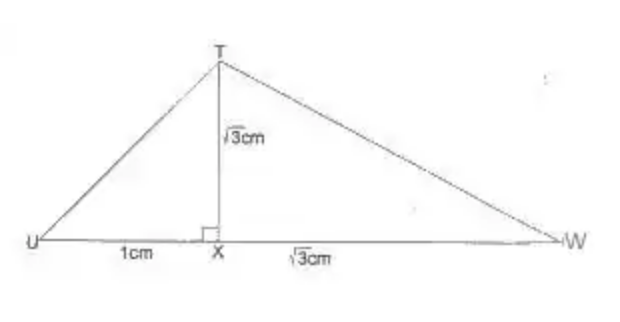In the diagram, TX is perpendicular to UW, |UX| = 1 cm and |TX| = |WX| = √3 cm. Find < UTW.

A) 135°

B) 105°

C) 75°

D) 60°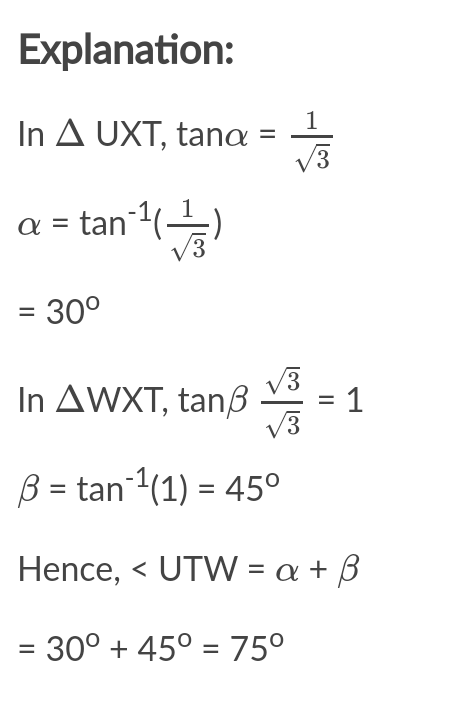30.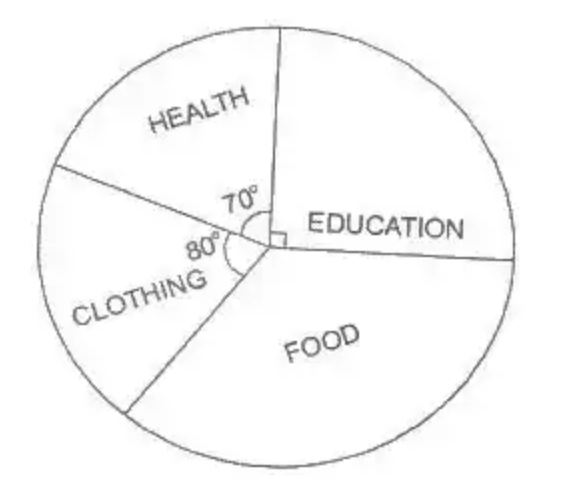The figure is a pie chart which represents the expenditure of a family in a year. If the total income of the family was Le 10,800,000.00, how much was spent on food?

A) Le 2,250,000.00

B) Le 2,700,000.00

C) Le 3,600,000.00

D) Le 4,500,000.00

Explanation:

sectoral angle representing food

= 360° – (80 + 70 + 90)°

= 120°

Amount spent on food

= \tectsectoralangle/360° x Le 10,800,000

= Le 3,600,000

31. A fair die is thrown two times. What is the probability that the sum of the scores is at least 10?

A) 536

B) 16

C) 518

D) 23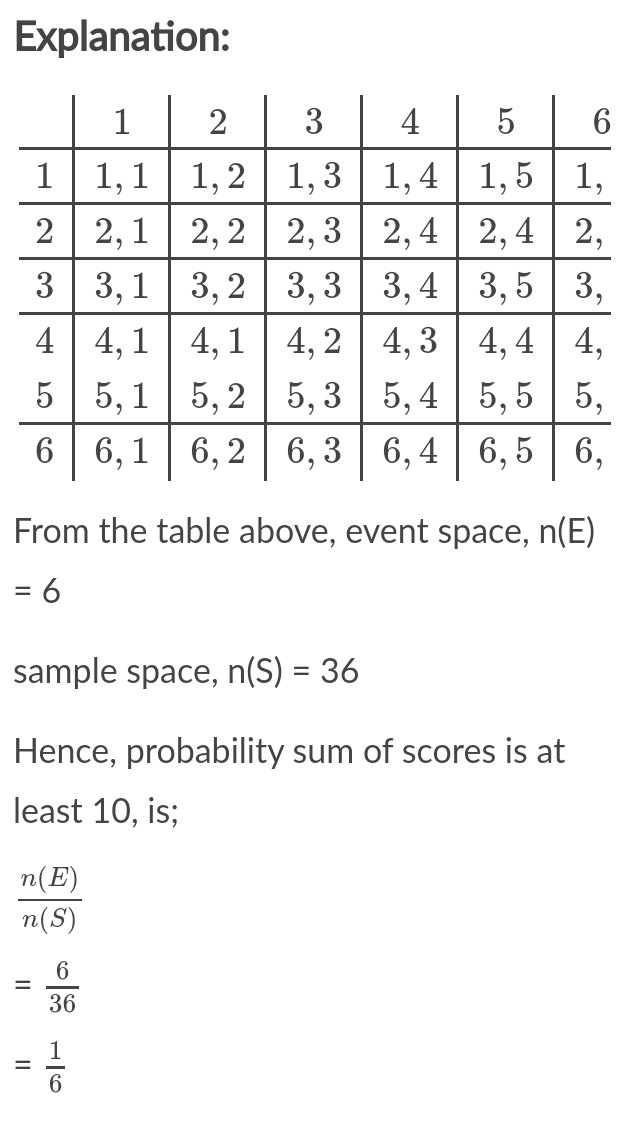32. The marks of eight students in a test are: 3, 10, 4, 5, 14, 13, 16 and 7. Find the range

A) 16

B) 14

C) 13

D) 11

Explanation:

First, arrange the marks in order of magnitude; 3, 4, 5, 7, 10, 13, 14, 16

Hence range = 16 – 3 = 13

32. If log_2(3x – 1) = 5, find x.

A) 2.00

B) 3.67

C) 8.67

D) 11.00

Explanation:

Log_2(3x – 1) = 5

Log_2(3x – 1) = Log_22⁵

Log_2(3x – 1) = Log_23²

3x – 1 = 32

3x = 32 + 1 = 33

x = 33/3

= 11

34. A sphere of radius r cm has the same volume as cylinder of radius 3 cm and height 4 cm. Find the value of r

A) 2/3

B) 2

C) 3

D) 6

Explanation:

Volume of sphere = Volume of cylinder

i.e. 4/3πr³ = πr²h

4/3πr³= π × 3² ×4

r³ = π × 9 × 4 × 3 / 4π

r = 3√27

= 3

35. Express 1975 correct to 2 significant figures

A) 20

B) 1,900

C) 1,980

D) 2,000

36.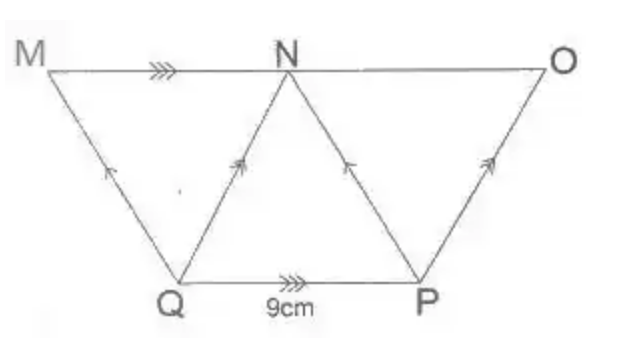The diagram, MOPQ is a trapezium with QP||MO, MQ||NP, NQ||OP, |QP| = 9 cm and the height of ΔQNP = 6 cm, calculate the area of the trapezium.

A) 96cm²

B) 90cm²

C) 81cm²

D) 27cm²

Explanation:

Area of Δ QNP = 1/2 × 9 × 6= 27cm2

Area of Δ QMN = Area of Δ QNP

= Area of Δ PNO (triangles between the same parallels)

Hence, area of the trapezium

3 x area of Δ QNP

= 3 x 27

= 81cm²

37. The perimeter of a sector of a circle of radius 21 cm is 64 cm. Find the angle of the sector [Take π=22/7]

A) 70°

B) 60°

C) 55°

D) 42°

Explanation:

Perimeter of a sector

= 2r + θ/360° x 2 x 22/7 x 21

64 = 2 x 21 + θ/360° x 2 x 22/7 x 21

64 = 42 + θ/360° x 44 x 3

64 – 42 = θ/360° x 11 x 3

22 = 33θ/90

θ=22×30/11

= 60°

38.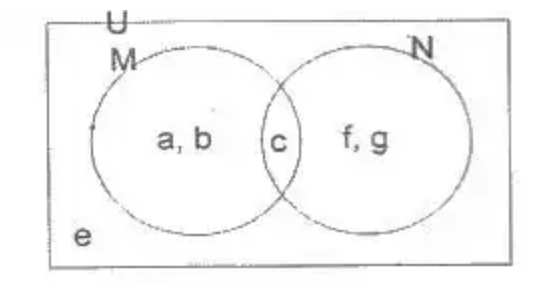Examine M’ ∩ N from the venn diagram.

A) {f, g}

B) {e}

C) {c, f, g}

D) {e, f, g}

Explanation:

From the venn diagram given,

M = (a, b, c), N = (c, f, g)

U = (a, b, c, d, e, f, g)

Thus M’ ∩ N = (e, f, g) ∩ (c, f, g)

= (f, g)

39. If 20(mod 9) is equivalent to y(mod 6), find y.

A) 1

B) 2

C) 3

D) 4

Explanation:

First, reduce 20(mod 9) to its simplest form in mod 9; 9 x 2 + 2 = 2(mod 9)

If 2(mod 9) y(mod 6), then y = 2 by comparism

40. Simplify (p−r)² − r² / 2p² − 4pr

A) 1/2

B) p – 2r

C) 1/p−2r

D) 2p/p−2r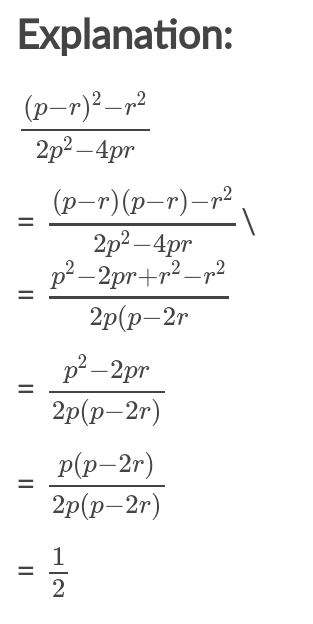41.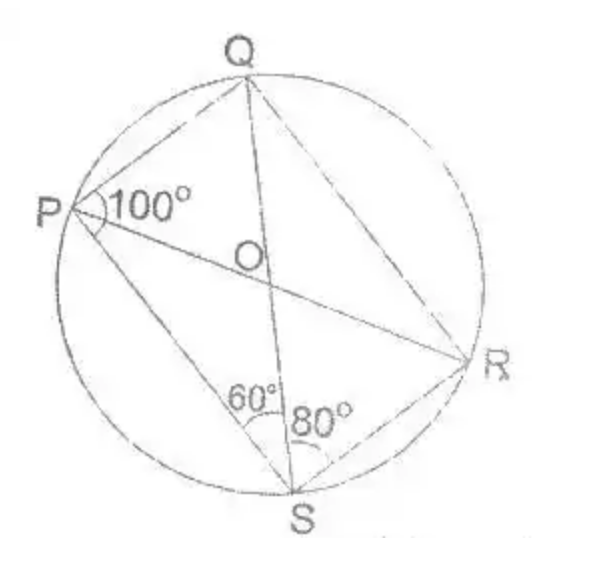In the diagram, O is the centre of the circle, < QPS = 100°, < PSQ = 60° and < QSR = 80°. Calculate < SQR.

A) 20°

B) 40°

C) 60°

D) 80°

Explanation:

In the diagram, < RPQ = 80°(angles in same segment)

< SPR = 100° – < RPQ

= 100 – 80

= 20°

< SQR = < SPR = 20° (same reason as above)

< SQR = 20°

42. A bag contains 5 red and 4 blue identical balls. Id two balls are selected at random from the bag, one after the other, with replacement, find the probability that the first is red and the second is blue

A) 2/9

B) 51/8

C) 20/81

D) 5/9

Explanation:

n(red balls) = 5

n(blue balls) = 4

n(⟺) = 9

Hence, prob (R_1, B_2)

= 5/9×4/9

= 20/81

Suggest Correction

43. Find the next three terms of the sequence; 0, 1, 1, 2, 3, 5, 8…

A) 13, 19, 23

B) 9, 11, 13

C) 11, 15, 19

D) 13, 21, 34

44.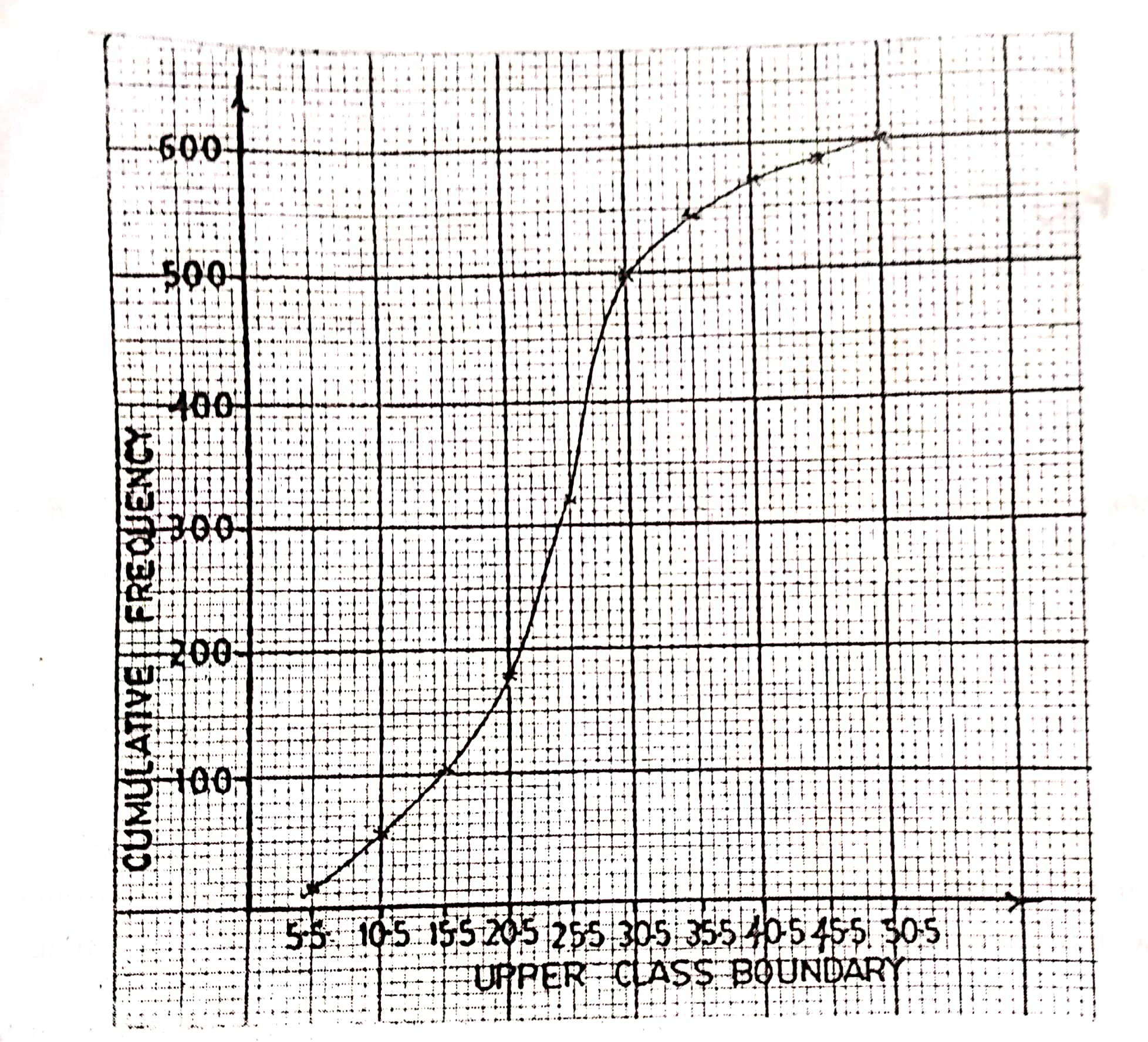Find the lower quartile of the distribution illustrated by the cumulative frequency curve.

A) 17.5

B) 19.0

C) 27.5

D) 28.0

Explanation:

Lower quartile,

Q_1 = 1/4Nth term

= 1/4 x 600th term

= 150th term

= 15.5 + 3 + 0.5

= 19

45. The ratio of the exterior angle to the interior angle of a regular polygon is 1:11. How many sides has the polygon?

A) 30

B) 24

C) 18

D) 12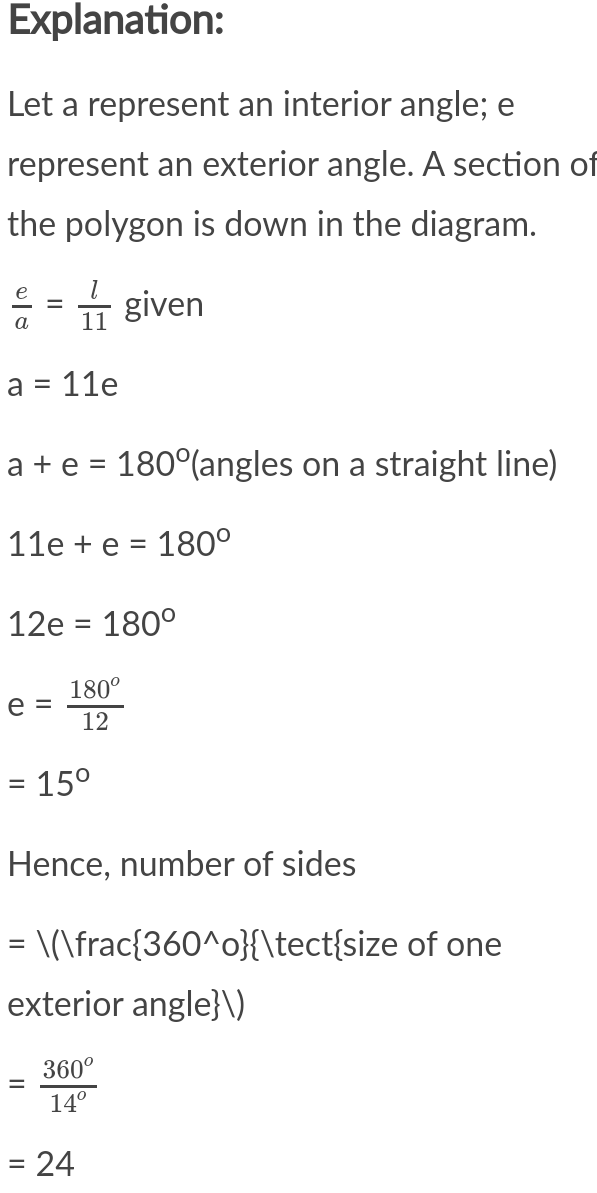46. Halima is n years old. Her brother’s age is 5 years more than half of her age. How old is her brother?

A) n/2+5/2

B) n/2 – 5

C) 5 – n/2

D) n/2 + 5

Explanation:

Halima’s age = n years old

Her brother’s age = n/2 + 5

47.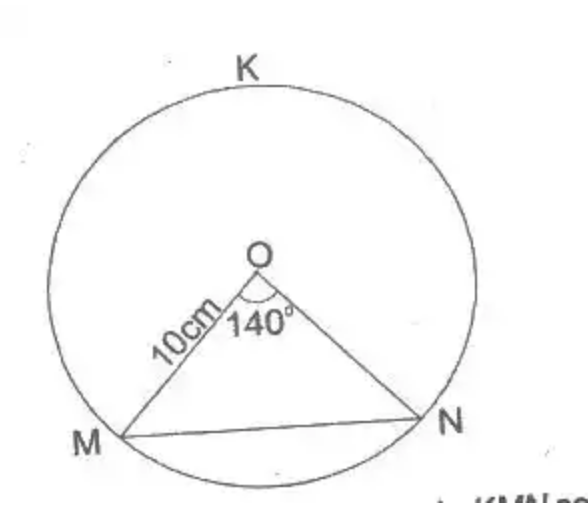In the diagram MN is a chord of a circle KMN centre O and radius 10 cm. If < MON = 140°, find, correct to the nearest cm, the length of the chord MN.

A) 19 cm

B) 18 cm

C) 17 cm

D) 12 cm

Explanation:

From the diagram

sin 70° = x/10

x = 10sin 70°

= 9.3969

Hence, length of chord MN = 2x

= 2 x 9.3969

= 18.7938

= 19cm (nearest cm)

48.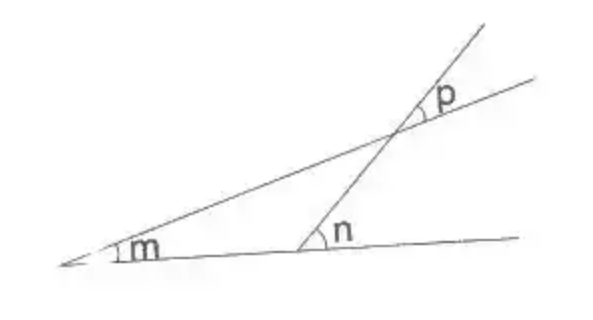From the diagram, which of the following is true?

A) m + n + p = 180°

B) m + n = 180°

C) m = p + n

D) n = m + p

Explanation:

In the diagram, β = p(vertically opposite angles)

m + β = n(sum of interior opp. angles)

m + p = n

49. Which of the following number lines illustrates the solution of the inequality 4=<1/3 (2x – 1) < 5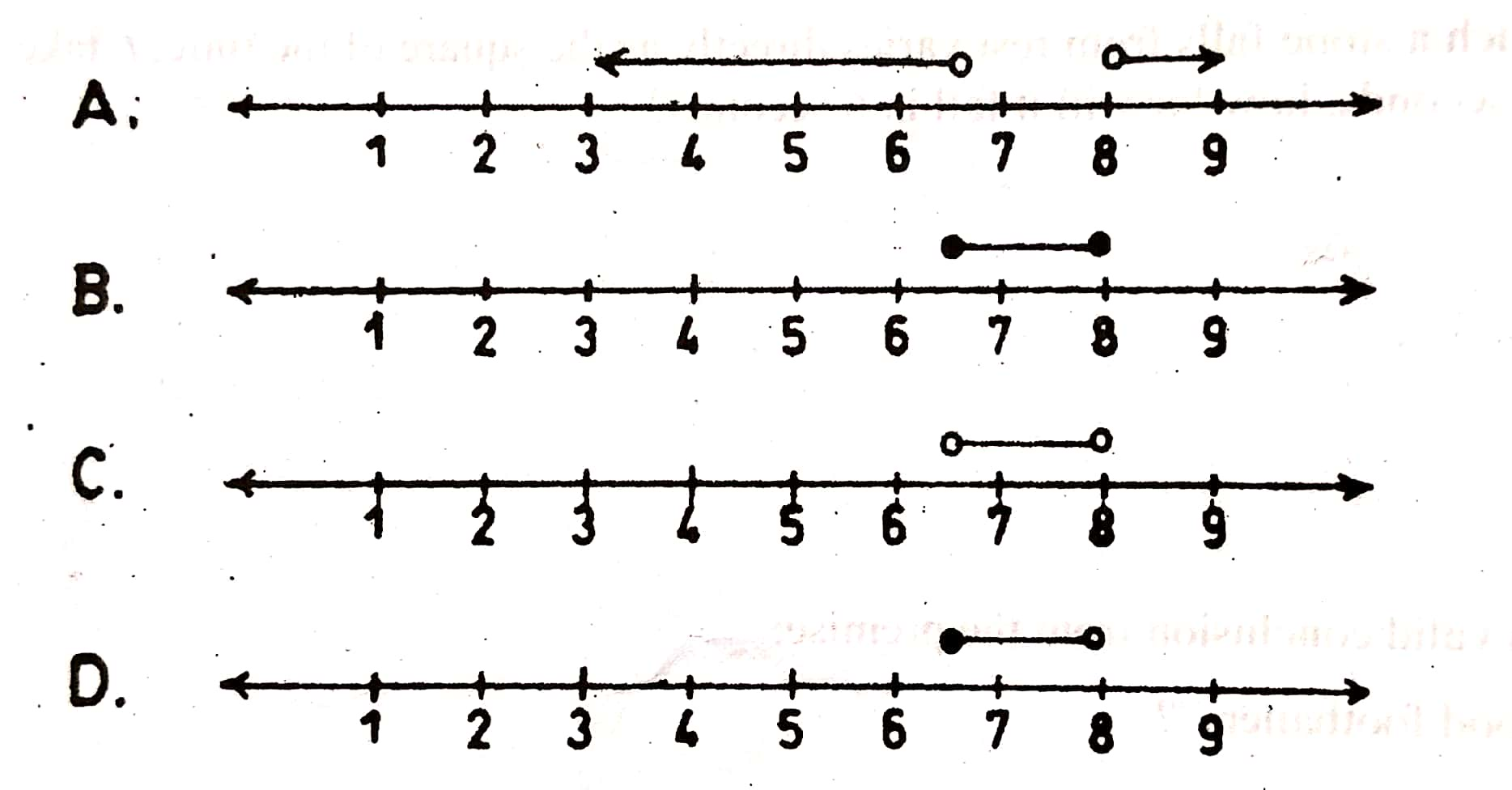50. Simplify: ( 3/4–2/3) × 1⅕

A) 1/60

B) 5/72

C) 1/10

D) 1(7/10)NGstudents: For Educational Information, Local And World Breaking News

1.FIKAYOR // __ August 12, 2020 at 8:00 PM // Reply
2.Chinedum Augustine // __ August 14, 2020 at 4:48 PM // Reply